Inverse Matrices
 Home > Lessons > Inverse Matrices Search | Updated March 23rd, 2019
Introduction

To calculate the inverse of a matrix, there are numerous details to consider. This lesson will provide insight to those details. Here are the sections within this lesson:

If you are not familiar with what a matrix is, please see MATHguide's lesson, called Matrices, before proceeding.

Imagine trying to solve this simple equation.Upon simple inspection, we can see that the value for 'x' has to be 1. Similarly, imagine this equation, where 'A' and 'X' are both matrices.Could there be such a matrix that when multiplied by another matrix it does not change the value of the first matrix? There is such a matrix and it is called the identity matrix. Common notation is to call the identity matrix 'I,' like so.You may have noticed the subscript value of '2.' The two indicates the size of the identity matrix. Since identity matrices are square matrices (which means the number of rows and column are the same), we need only use one number to describe the size of the identity matrix.

Let's take a look what the elements are of our identity matrix, which was labeled "I-sub-two," above. The subscript of two indicates it is a 2x2 matrix.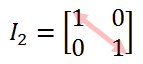Notice that the identity matrix has a diagonal of 1s that start at the top-left and proceed to the bottom right. The rest of the elements are 0s. This is true for larger identity matrices, such as "I-sub-3."If we were to take a 3x3 matrix, called matrix B, and multiply it times its identity matrix, we would get the matrix B back again. This is easily checked with an advanced calculator. We will not investigate the claim further within this lesson, however.

The determinant is a calculation that can be carried out on most square matrices. It can never be carried out on any other type of matrix but square matrices. Let us look at matrix C.If we wanted to find the determinant of matrix C, it could be written either of these ways.The "det" means "determinant of." Likewise, placing vertical lines around a matrix (without the a matrix typical brackets) is the most common way of depicting a determinant.

Now, let us see how it is we calculate a determinant. A 2x2 determinant is relatively easy to calculate -- compared to larger sized square matrices. We multiply both diagonals and subtract the products. We start from the top left to the bottom right, like so.Then, we multiply the other diagonal.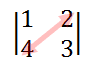Last, we subtract the products, like so.Let us look at another example, especially when there are elements that have negative values.Multiply the 6 and 4 first.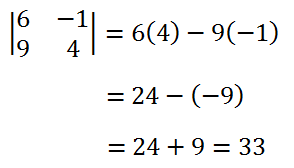uiz: Evaluating 2x2 Determinantsuiz: Evaluating 3x3 Determinants

Now that we know what an identity matrix is and how to calculate a determinant (at least a 2x2 determinant), we can calculate an inverse. First, let's get some notation straight. The inverse of a matrix is indicated by placing a -1 exponent either on the name of the matrix or the matrix itself.

If we wanted to talk about the inverse of matrix D, we could write it like this.If we knew this to be matrix D......we could write the inverse of matrix D like so.When using advanced calculators, this is exactly how we get the calculators to compute an inverse. Now, let's see how it is we calculate an inverse by hand. We will use a generic matrix to explain. Here is matrix E.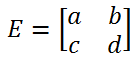To calculate the inverse, we need to follow this rule.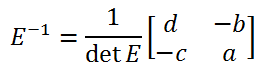Notice this: 1) we have to calculate the determinant of E and place it in a denominator, 2) the 'a' and the 'd' switched positions, and 3) the 'c' and 'd' did not move, but their values are now the opposite. Here is matrix D from above. We can use this to see how an inverse can be calculated on a specific matrix.Here is another example.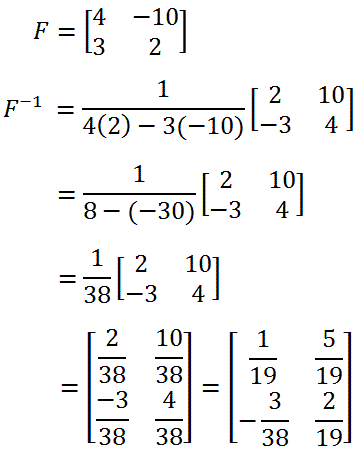Here is a video that will help you to understand how to determine the inverse of a matrix. It will explain crucial points, like what an identity matrix is, how to calculate determinants, and how to get an inverse both by hand and with a calculator.ideo: Inverse of a Matrix: Multiplicativeuiz: Inverse Matrices

Use this video to learn how to use a matrix equation to solve a system of linear equations.ideo: Matrix Equations

Try an activity related to the lessons above.ideo: Inverse of a Matrix: Multiplicativeideo: Matrix Equationsuiz: Evaluating 2x2 Determinantsuiz: Evaluating 3x3 Determinantsuiz: Inverse Matrices

Try an activity related to the lessons above.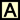ctivity: Matrix Equationsesson: Matrices